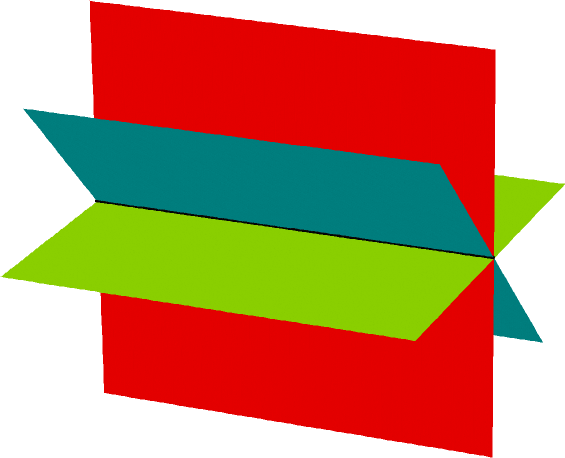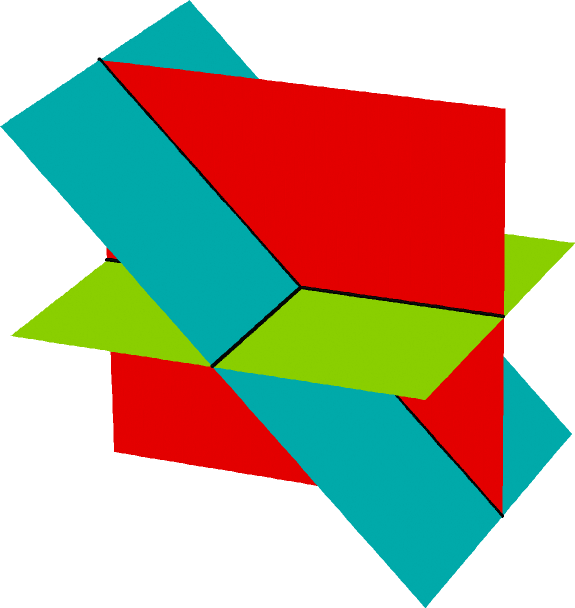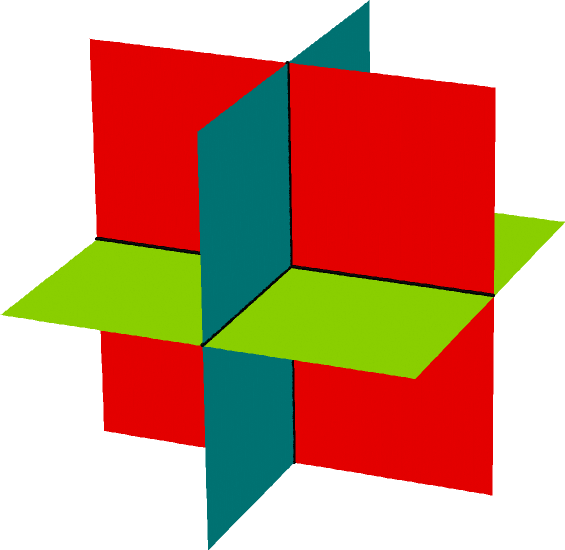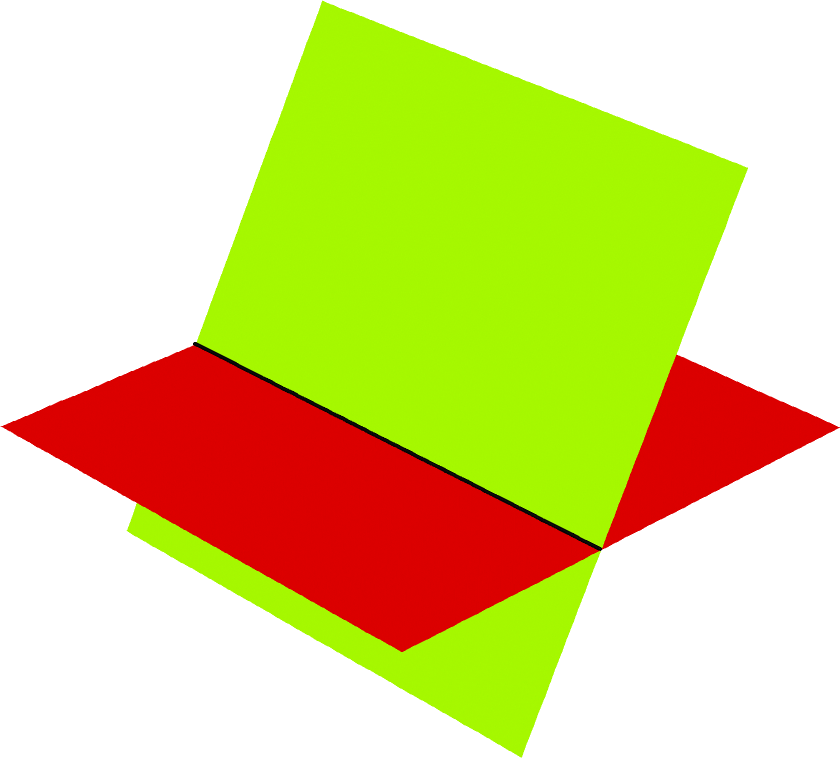Each graph below is the graph of a system of three linear equations in three unknowns of the form $A \vec{x} = \vec{b}$. Determine the rank of the matrix $A$.A. choose rank 1 rank 2 rank 3 B. choose rank 1 rank 2 rank 3 C. choose rank 1 rank 2 rank 3D. choose rank 1 rank 2 rank 3 E. choose rank 1 rank 2 rank 3

(Click on a graph to enlarge it.)

In order to get credit for this problem all answers must be correct.